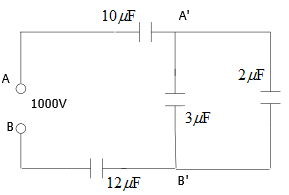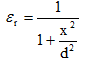# Important Problems on Capacitors and capacitance for JEE Main And Advanced

Question 1
A parallel plate air capacitors has plate area 0.2 m2 and has separation distance 5.5 mm. Find
(a) Its capacitance when capacitor is charged to a potential difference of 500 volts
(b) Its charge
(c) Energy stored in it
(d) Force of attraction between the plates
Question 2
Consider a system of capacitors where two parallel plate air capacitors each of capacitance C are connected in series to a battery of EMF ξ. Now one of the capacitor is filled uniformly with a dielectric of dielectric constant K. What would happen to electric field strength of that capacitor and what would be the change in electric field strength?  Calculate the amount of charge that flows through the battery?
Question 3
A spherical capacitor has charges + Q and - Q on its inner and outer conductors. Find the electric potential energy stored in the capacitor?
Question 4
Find the capacitance of an isolated spherical conductor of radius r1 surrounded by an adjacent concentric layer of dielectric with dielectric constant K and outside radius r2.
Question 5
In a parallel plate air capacitor having plate separation 0.05mm, an electric field of 4x104 V/m is established between the plates. After the removal of the battery a metal plate of thickness t=0.02mm is inserted between the plates of the capacitor. Find
1. Potential difference across capacitor before the introduction of metal plates.
2. Potential difference across capacitor after the introduction of metal plates.
3. Potential difference across capacitor if dielectric slab with dielectric constant K=3 and same thickness were inserted in place of metal plate.
Question 6
Find the capacitance of four parallel plates each of area Am2 and separated by a distance d1, d2, and d3 . The space between them is filled with dielectrics of relative permittivity ε1, ε2 and ε3 . Permittivity of free space is ε0.
Question 7
Consider the figure given below1. Find the equivalent capacitance between A and B
2. Find the potential difference between 3μF capacitor
3. Find the amount of charge on 2μF capacitor
4. Find the energy stored on 12μF capacitor
Question 8
Two parallel plate condensers A and B having capacities 2 μF and 10 μF are charged separately to the same potential of 200V. Now, positive plate of  A is connected to negative plate of B and the negative plate of A is connected to positive plate of B.
1. Calculate the equivalent capacitance and the common voltage of combination A-B.
2. Find the loss in electrical energy in each condenser.
Question 9
The plates of a parallel plate capacitor are separated by a dielectric whose relativity permittivity varies according to following relationFind the capacitance of the capacitor.

Solutions